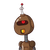# if the rate of 1kg iron junk is ₹25 then how much money is earned from 65 kg iron junk?​

if the rate of 1kg iron junk is ₹25 then how much money is earned from 65 kg iron junk?​

### 1 thought on “if the rate of 1kg iron junk is ₹25 then how much money is earned from 65 kg iron junk?​”

1.## ■Solution :

The rate of 1kg iron junk is ₹25.

:. the rate of 65kg of iron junk= 65×₹25

= ₹1625

## ● Answer: ₹1625 is the rate of 65 kg iron junk.

Hope this helps you!